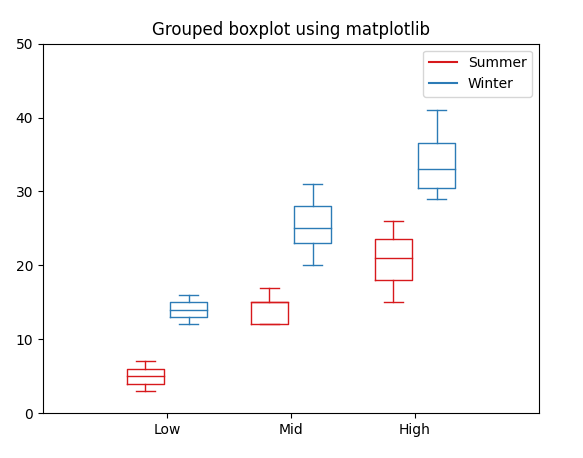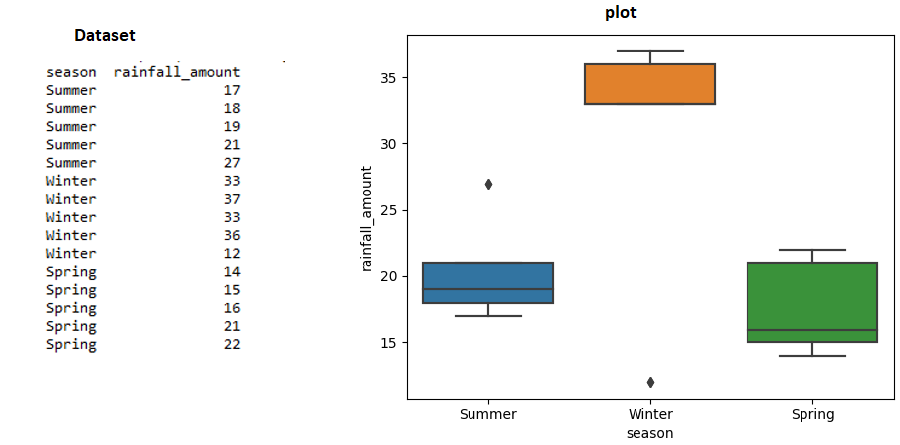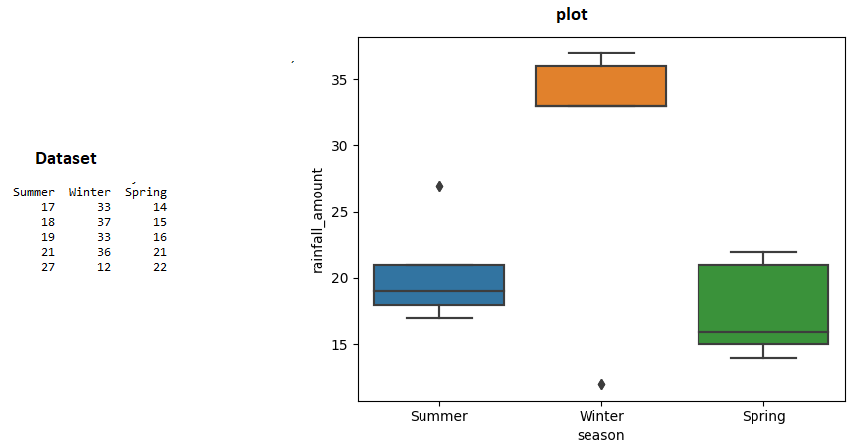# How to Create Boxplots by Group in Matplotlib?

• Last Updated : 25 Jan, 2022

Boxplots by groups can be created using the matplotlib package, but, however, if you wish to make more customizations to your grouped box plot, then the seaborn package provides a go-to function that supports a wide variety of customizations to the grouped box plots. Matplotlib doesn’t provide an explicit function to create a grouped box plot. We have to construct the plot as per the required format. This article discusses how to create grouped boxplots in matplotlib.

## Create Boxplots by Group in Matplotlib

matplotlib.pyplot.boxplot() & matplotlib.pyplot.setp() are the two useful functions to create grouped boxplots

Syntax: matplotlib.pyplot.boxplot(x, notch, positions, widths)

Syntax: matplotlib.pyplot.setp(obj, *args, **kwargs)

## Python3

 `# import the matplotlib package``import` `matplotlib.pyplot as plt` `# import the numpy package``import` `numpy as np` `# create 2 - sample a 3-Dim array, that measures``# the summer and winter rain fall amount``summer_rain ``=` `[[``3``, ``5``, ``7``], [``15``, ``17``, ``12``, ``12``, ``15``],``               ``[``26``, ``21``, ``15``]]``winter_rain ``=` `[[``16``, ``14``, ``12``], [``31``, ``20``, ``25``, ``23``, ``28``],``               ``[``29``, ``31``, ``35``, ``41``]]` `# the list named ticks, summarizes or groups``# the summer and winter rainfall as low, mid``# and high``ticks ``=` `[``'Low'``, ``'Mid'``, ``'High'``]` `# create a boxplot for two arrays separately,``# the position specifies the location of the``# particular box in the graph,``# this can be changed as per your wish. Use width``# to specify the width of the plot``summer_rain_plot ``=` `plt.boxplot(summer_rain,``                               ``positions``=``np.array(``    ``np.arange(``len``(summer_rain)))``*``2.0``-``0.35``,``                               ``widths``=``0.6``)``winter_rain_plot ``=` `plt.boxplot(winter_rain,``                               ``positions``=``np.array(``    ``np.arange(``len``(winter_rain)))``*``2.0``+``0.35``,``                               ``widths``=``0.6``)` `# each plot returns a dictionary, use plt.setp()``# function to assign the color code``# for all properties of the box plot of particular group``# use the below function to set color for particular group,``# by iterating over all properties of the box plot``def` `define_box_properties(plot_name, color_code, label):``    ``for` `k, v ``in` `plot_name.items():``        ``plt.setp(plot_name.get(k), color``=``color_code)``        ` `    ``# use plot function to draw a small line to name the legend.``    ``plt.plot([], c``=``color_code, label``=``label)``    ``plt.legend()`  `# setting colors for each groups``define_box_properties(summer_rain_plot, ``'#D7191C'``, ``'Summer'``)``define_box_properties(winter_rain_plot, ``'#2C7BB6'``, ``'Winter'``)` `# set the x label values``plt.xticks(np.arange(``0``, ``len``(ticks) ``*` `2``, ``2``), ticks)` `# set the limit for x axis``plt.xlim(``-``2``, ``len``(ticks)``*``2``)` `# set the limit for y axis``plt.ylim(``0``, ``50``)` `# set the title``plt.title(``'Grouped boxplot using matplotlib'``)`

Output:Explanation:

• Import the necessary packages numpy and matplotlib.
• Create 2 – sample arrays of 3 dimensions named, summer_rain and winter_rain
• Create another list named ticks, that summarizes or groups the summer and winter rainfall as low, mid, and high.
• Create a boxplot for two arrays separately as shown.
• Use the position argument to specify the location of every box in the group, here, the summer_rain plot has 3 boxes which are separated at a spacing of [-0.35,  1.65,  3.65] and the winter_rain plot has 3 boxes which are separated at a spacing of [0.35, 2.35, 4.35].
• The width of each box is kept at 0.6.
• Now, each individual plot summer_rain_plot and winter_rain_plot returns a dictionary, This dictionary has a list of properties of the box plot like whiskers, median, fliers etc.
• Now, iterate through the dictionary items and use plt.setp() function to assign a unique color code for each group as shown.
• Use plt.plot() function to draw a default line to represent the legends of the box plot.
• The define_box_properties function, takes the plot, color and the legend name as arguments and set the properties of the plot appropriately.
• Finally, to improve the aesthetic value, use xlim, ylim function to define the limits of the x and y axis and use xticks function to label the x-axis. Set the title of the plot using plt.title() function.

## Create Boxplots by Group in seaborn

You can also plot grouped box plots using long-form and wide form data using yet another library called seaborn which is built on top matplotlib library.

Syntax: sns.boxplot(data, x, y)

Parameters:

• data – specifies the dataframe to be used for the box plots
• x –  specifies the column to be used in the x-axis
• y – specifies the column to be used in y-axis

## Python3

 `# import the necessary python packages``import` `pandas as pd``import` `numpy as np``import` `seaborn as sns` `# create long-form data``data ``=` `pd.DataFrame({``'season'``: np.repeat([``'Summer'``, ``'Winter'``,``                                          ``'Spring'``], ``5``),``                     ``'rainfall_amount'``: [``17``, ``18``, ``19``, ``21``, ``27``,``                                         ``33``, ``37``, ``33``, ``36``, ``12``,``                                         ``14``, ``15``, ``16``, ``21``, ``22``],``                     ``})``# print the data``print``(data)` `# use seaborn plot and specify the x and y``# columns and specify the dataframe``sns.boxplot(x``=``'season'``, y``=``'rainfall_amount'``, data``=``data)`

Output:## Python3

 `# import the necessary python packages``import` `pandas as pd``import` `numpy as np``import` `seaborn as sns` `# create wide-form data``data ``=` `pd.DataFrame({``'Summer'``: [``17``, ``18``, ``19``, ``21``, ``27``],``                     ``'Winter'``: [``33``, ``37``, ``33``, ``36``, ``12``],``                     ``'Spring'``: [``14``, ``15``, ``16``, ``21``, ``22``]})``# print the data``print``(data)``# use melt to convert wide form to long form data``# use seaborn plot and specify the x and y columns``# and specify the dataframe``sns.boxplot(x``=``'variable'``, y``=``'value'``, data``=``pd.melt(data)).``set``(``    ``xlabel``=``'Season'``,``    ``ylabel``=``'Rainfall amount'``)`

Output:Code Explanation:

• Import the necessary packages
• Create a sample dataframe that lists seasonal rainfall amounts in wide form format as shown.
• To plot the grouped box plot, the data has to be in a long format, so use pandas.melt() function to melt the data from the wide form to long-form.
• When the wide form data is converted to long-form data, the two columns will be named as ‘variable’ and ‘value’ by default.
• Use seaborn plot and pass the ‘variable’  as x and ‘value’ as y column of the boxplot and the corresponding dataframe.
• Use the set() function to set the x and y-axis labels of the boxplot.

My Personal Notes arrow_drop_up